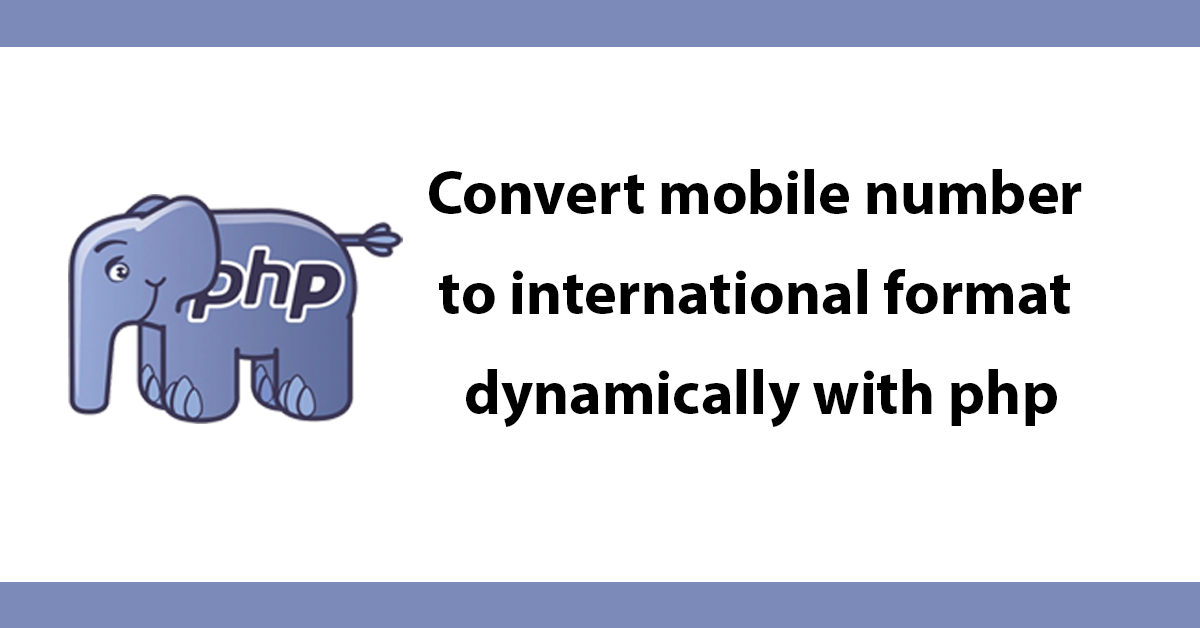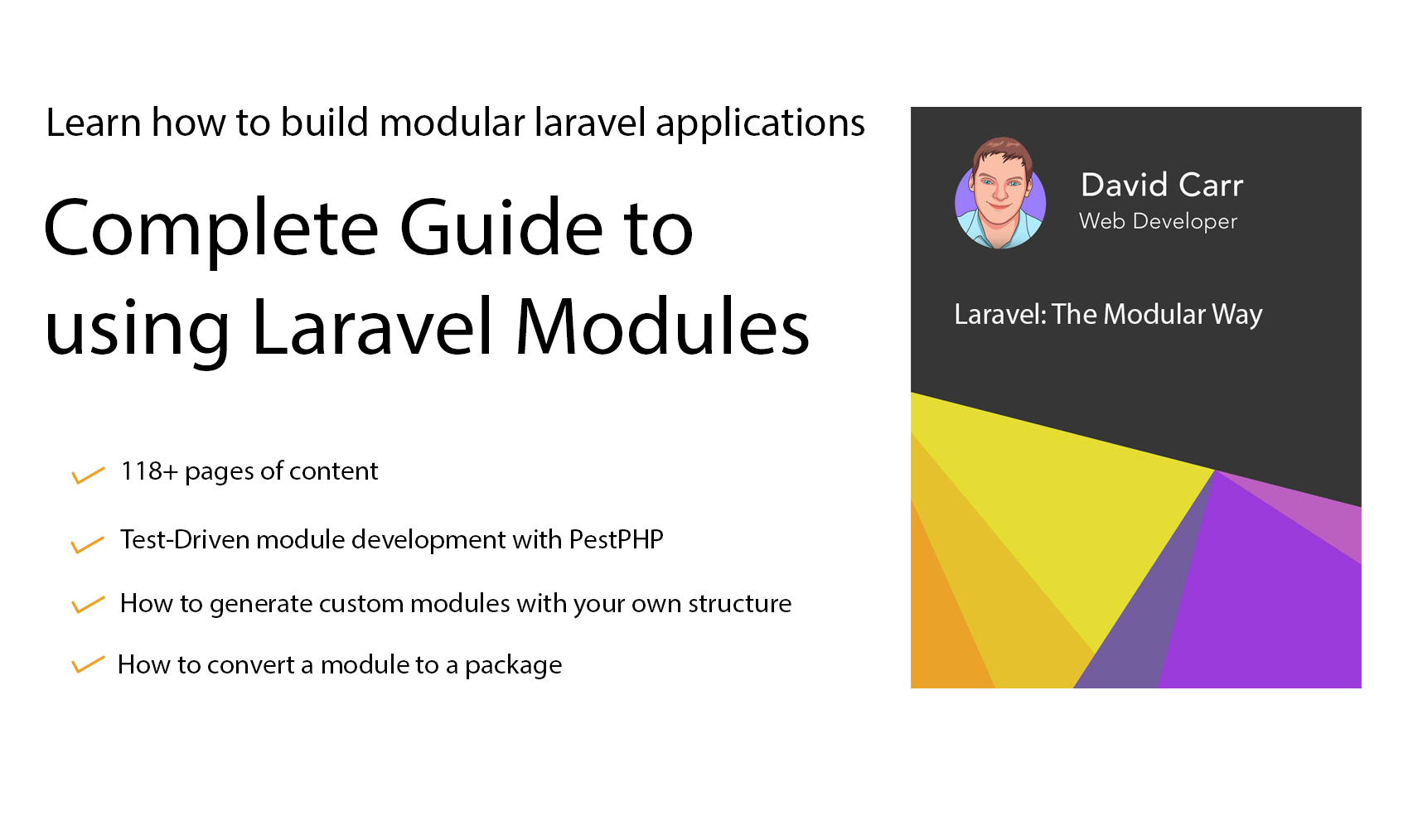# Convert mobile number to international format dynamically with php

I often need to covert mobile numbers into a UK international format of 44 followed by the number such as 442548951486 the numbers I work with can be in a mixed format some without a starting 0 others with 44 and same with spaces, so I wrote function that will convert a number in any mixture into a standard format quick and easily and as all the code is inside a function is reusable which means converting numbers in bulk is now very easy to do.

The function simple needs a number passing to it, a check is done to make sure the number only contains numbers then a check is done to see if the number starts with a 0 if not if will add a 0 as long as the start is not 4. Then a check is done to replace the 0 with a 44 then finally any spaces are removed and the formatted number is then returned.

Here's the function:

``````<?php
function format_number(\$number)
{
//make sure the number is actually a number
if(is_numeric(\$number)){

if(\$number != 0 && \$number != 4){
\$number = "0".\$number;
}

//if number starts with a 0 replace with 4
if(\$number == 0){
\$number = str_replace("0","4",\$number);
\$number = "4".\$number;
}

//remove any spaces in the number
\$number = str_replace(" ","",\$number);

//return the number
return \$number;

//number is not a number
} else {

//return nothing
return false;
}
}
?>``````

<p>Usage Example:</p>

``echo format_number('07295514973');``Help support the blog so that I can continue creating new content!